OpenFOAM 成长之路 2021-01-13T00:00:00.000Z https://openfoam.top/ Yan Zhang Hexo VS Code + LSP in OpenFOAM https://openfoam.top/vscodeLSP/ 2021-01-13T00:00:00.000Z 2021-01-13T00:00:00.000Z 使用 VS Code + Language Server Protocol 更好地阅读以及编写 OpenFOAM 代码。

]]>
<p>使用 VS Code + Language Server Protocol 更好地阅读以及编写 OpenFOAM 代码。</p>
FlameMaster 编译教程 https://openfoam.top/FlameMaster/ 2021-01-04T00:00:00.000Z 2021-01-04T00:00:00.000Z FlameMaster 编译教程

# 开始使用

]]>
<p>FlameMaster 编译教程</p>
VS Code 远程调试 OpenFOAM https://openfoam.top/vscodeDebug/ 2020-12-12T00:00:00.000Z 2021-01-05T00:00:00.000Z 使用 VS Code 调试远程服务器上的 OpenFOAM 代码。

# 方法一

.vscode/c_cpp_properties.json

.vscode/tasks.json

.vscode/launch.json

.vscode/settings.json

# 方法二

.vscode/c_cpp_properties.json

.vscode/launch.json

.vscode/settings.json

]]>
<p>使用 VS Code 调试远程服务器上的 OpenFOAM 代码。</p>
OpenFOAM 功能探索：stopAt https://openfoam.top/stopAt/ 2020-08-16T00:00:00.000Z 2020-08-16T00:00:00.000Z 通过 stop 文件 或者 运行时间 终止 OpenFOAM 算例

## stopAtClockTime

### 用途举例

]]>
<p>通过 <strong>stop 文件</strong> 或者 <strong>运行时间</strong> 终止 OpenFOAM 算例</p>
OpenFOAM 并行 reconstructPar https://openfoam.top/parallellyReconstructPar/ 2020-07-17T00:00:00.000Z 2021-02-05T00:00:00.000Z 使用特殊技巧并行化 reconstructPar OpenFOAM 结果

]]>
<p>使用特殊技巧并行化 reconstructPar OpenFOAM 结果</p>
paraview 网格显示美化 https://openfoam.top/paraviewMesh/ 2020-07-08T00:00:00.000Z 2020-07-08T00:00:00.000Z paraview 的网格可以显示得很美观，只需要掌握以下技巧。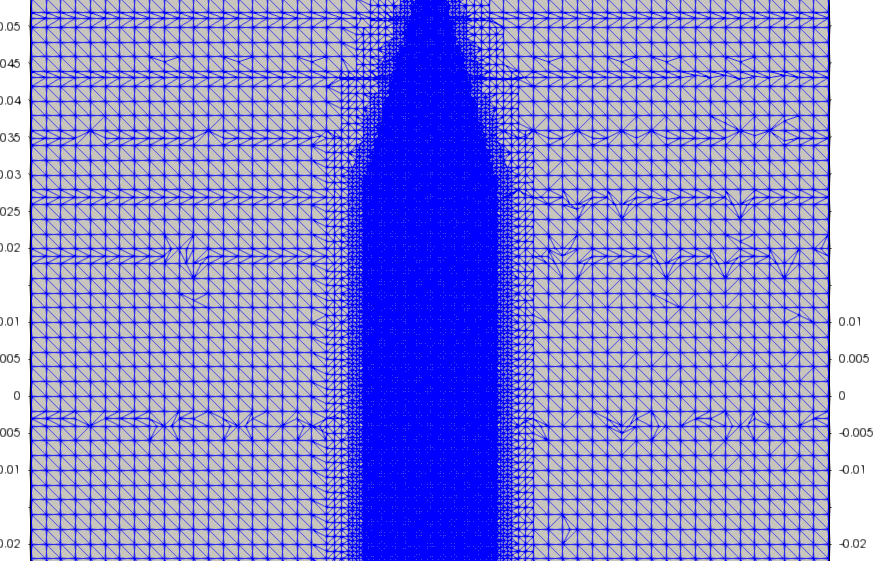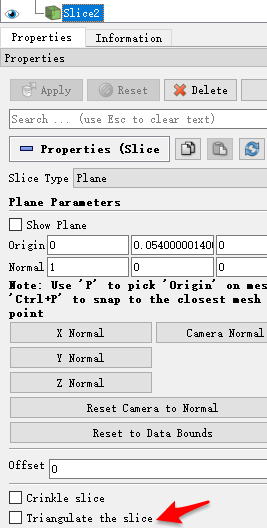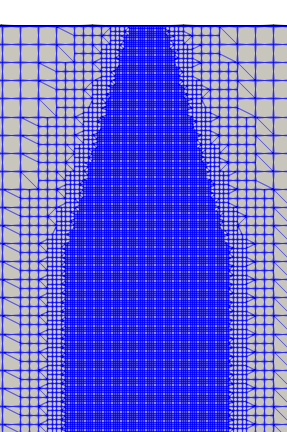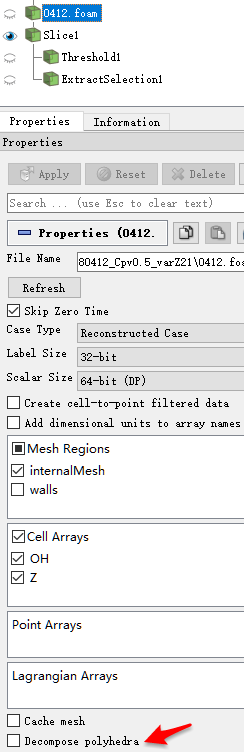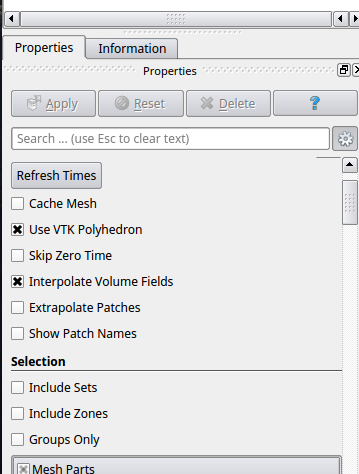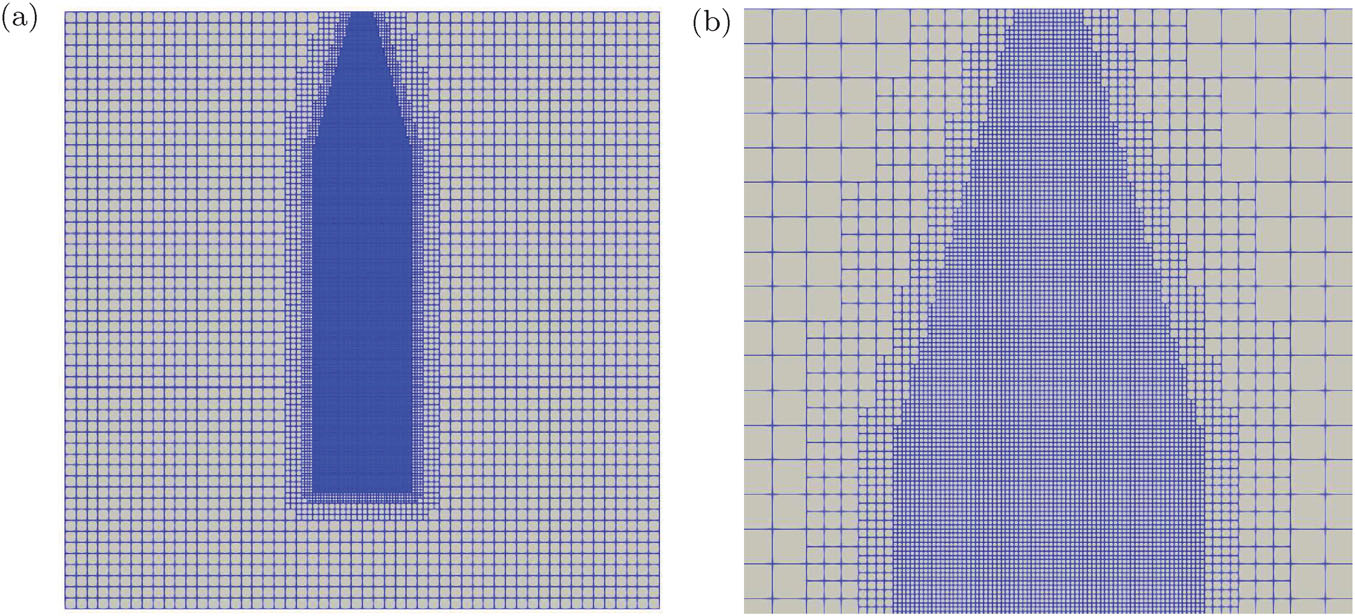]]>
<p>paraview 的网格可以显示得很美观，只需要掌握以下技巧。</p>
OpenFOAM with boost https://openfoam.top/boost/ 2020-06-02T00:00:00.000Z 2020-08-20T00:00:00.000Z using boost in OpenFOAM

# boost 中的函数

beta(a,b)$\int_0^1t^{a-1}(1-t)^{b-1}dt=\dfrac{\Gamma(a)\Gamma(b)}{\Gamma(a+b)}$
beta(a,b,x)$\int_0^xt^{a-1}(1-t)^{b-1}dt$
ibeta(a,b,x)$\dfrac{\int_0^xt^{a-1}(1-t)^{b-1}dt}{\int_0^1t^{a-1}(1-t)^{b-1}dt}=\dfrac{beta(a,b,x)}{beta(a,b)}$

# GSL 中的函数

gsl_sf_beta(a,b)$\int_0^1t^{a-1}(1-t)^{b-1}dt=\dfrac{\Gamma(a)\Gamma(b)}{\Gamma(a+b)}$
gsl_sf_beta_inc(a,b,x)$\dfrac{\int_0^xt^{a-1}(1-t)^{b-1}dt}{\int_0^1t^{a-1}(1-t)^{b-1}dt}$
]]>
<p>using boost in OpenFOAM</p>
OpenFOAM with GSL https://openfoam.top/gsl/ 2020-06-02T00:00:00.000Z 2020-06-02T00:00:00.000Z using GSL (GNU Scientific Library) in OpenFOAM

# 系统环境变量

~/.bashrc 文件中添加：

# 在 OF solver 中使用 GSL

options 文件中添加：

# 使用举例

## 矩阵相乘

]]>
<p>using GSL (GNU Scientific Library) in OpenFOAM</p>
OpenFOAM with OpenMP https://openfoam.top/openmp/ 2020-04-08T00:00:00.000Z 2020-04-08T00:00:00.000Z using OpenMP in OpenFOAM

]]>
<p>using OpenMP in OpenFOAM</p>
OpenFOAM中圆柱体的二维画法 https://openfoam.top/axialMesh/ 2019-10-08T00:00:00.000Z 2020-03-29T00:00:00.000Z 本文移植自博主以前的新浪博客，原文发表于 (2014-12-25 10:22:36)。

# 操作步骤

• 把需要坍塌的那个面（此面合并成一条线，即圆柱体的轴线），定义为center ， type symmetryPlane（定义边界条件是也用此type）。
• 需要frontAndBack。
• 生成楔形网格后，frontAndBack会变成两个 frontAndBack_pos， frontAndBack_neg。定义边界条件时，用wedge类型。

# 注意事项

• 只有一个网格的那个维度，需要与 0 对称分布：

• 如果在执行 collapseEdges -overwrite 的时候遇到以下问题：

可以执行以下代码，然后 goto 这里重新操作一遍！

• 如果使用 OF-2.1.x，需要使用： collapseEdges -overwrite 1e-6 179

# 相关链接

cfd-online

]]>
<p>本文移植自博主以前的新浪博客，<a href="http://blog.sina.com.cn/s/blog_a2a8de490102vbvh.html">原文</a>发表于 (2014-12-25 10:22:36)。</p>
OpenFOAM中refineMesh的用法 https://openfoam.top/refineMesh/ 2019-10-08T00:00:00.000Z 2020-04-11T00:00:00.000Z 本文移植自博主以前的新浪博客，原文发表于 (2014-12-25 09:58:12)。

https://github.com/OpenFOAM/OpenFOAM-dev/tree/master/etc/caseDicts/mesh/manipulation/refineRegion

]]>
<p>本文移植自博主以前的新浪博客，<a href="http://blog.sina.com.cn/s/blog_a2a8de490102vbve.html">原文</a>发表于 (2014-12-25 09:58:12)。</p>
OpenFOAM 中的喷雾子模型 https://openfoam.top/spraySubModels/ 2019-08-04T00:00:00.000Z 2019-08-04T00:00:00.000Z 从数学公式到 C++ 代码

# dispersion

## 传统 RANS 框架下的公式

\begin{aligned} \frac{d}{dt} \mathbf{x}_{\mathbf{p}} &=\mathbf{u}_{\mathbf{p}} \\ \frac{d}{d t} \mathbf{u}_{\mathbf{p}} &=\frac{C_{D}}{\tau_{p}} \frac{R e_{p}}{24}\left(\mathbf{u}_{\mathbf{g}}-\mathbf{u}_{\mathbf{p}}\right)=\frac{C_{D}}{\tau_{p}} \frac{R e_{p}}{24} \mathbf{v}_{\mathbf{r e l}} \end{aligned}

$\begin{array}{ll}C_D=\dfrac{24}{Re_p}\left(1+\dfrac{1}{6} Re_p^{2/3}\right) & R e_{p}<1000 \\ C_D=0.424 & Re_p \geqslant 1000\end{array}$

$R e_{p}=\rho_{g}\left|\mathbf{v}_{\mathrm{rel}}\right| d_{p} / \mu_{g}$

$\mathbf{v}_{\mathrm{rel}}=\tilde{\mathbf{u}}+\mathbf{u}^{\prime}-\mathbf{u}_{\mathrm{p}}$

$\tau_{p}=\rho_{l} d_{p}^{2} / 18 \rho_{g} v_{g}$

$\tilde{\mathbf{u}}$ 是气相速度，$\mathbf{u}^{\prime}$ is stochastic velocity vector accounting for turbulence dispersion of parcels via interaction with surrounding gases.
$\mathbf{u}^{\prime}$ 是高斯分布，均值等于 0，方差等于 $\sigma^2=2 k / 3$

In this way each component of $\mathbf{u}^{\prime}$ is chosen randomly from the Gaussian distribution

$G\left(\mathbf{u}_{\mathbf{p}, \mathbf{i}}^{\prime}\right)=\frac{1}{\sigma \sqrt{2 \pi}} \exp \left(-\frac{\mathbf{u}_{\mathbf{p}, \mathbf{i}}^{\prime}}{2 \sigma}\right)^{2}$

$t_{t u r b}=\min \left(\frac{k}{\varepsilon}, C_{p s} \frac{k^{3 / 2}}{\varepsilon} \frac{1}{\left|\tilde{\mathbf{u}}+\mathbf{u}^{\prime}-\mathbf{u}_{p}\right|}\right)$

OF 中的 stochstic turbulence dispersion 模型代码

KinematicParcel::move 中调用了 p.calcDispersion(cloud, ttd, dt);

## 针对 LES 的 dispersion 模型

Tsang C-W, Kuo C-W, Trujillo M, et al. Evaluation and validation of large-eddy simulation sub-grid spray dispersion models using high-fidelity volume-of-fluid simulation data and engine combustion network experimental data[J]. International Journal of Engine Research, 2019, 20(6):583–605.

$t_{turb}=C_{turb} \frac{2 \Delta}{\left|\mathbf{u}_{S g S}-\mathbf{u}_{d}\right|}$

$\mathbf{u}^{\prime}=\mathbf{u}_{sgs}+\mathbf{u}_{s t o}$

$\mathbf{u}_{s g s}=C_{s g s}(2 \tilde{\mathbf{u}}-3 \widetilde{\tilde{\mathbf{u}}}+\widetilde{\tilde{\mathbf{u}}})$

$\mathbf{u}_{sto}$ 是高斯分布，均值是0，方差是 $\sigma_{s g s}^{2}=C_{sig} \frac{2}{3} k_{s g s}$，其中 $C_{sig}$ 是模型参数。

$G\left(\mathbf{u}_{sto, i}\right)=\frac{1}{\sqrt{2 \sigma_{s g s}^{2} \pi}} \exp \left(-\frac{\mathbf{u}_{sto, i}^{2}}{2 \sigma_{s g s}^{2}}\right)$

# drag

CdRe：

calcCoupled：

SphereDragForce::calcCoupled 的返回值翻译成公式就是 $\dfrac{3m_p\mu_g C_DRe}{4\rho_p D^2}$

$F_p = m_p \dfrac{d \mathbf{u}_p}{d t}=\dfrac{3m_p\mu_gC_DRe}{4\rho_p D^2}(\mathbf{u}-\mathbf{u}_{p})$

SphereDragForce::calcCoupled 返回值即 $(\mathbf{u}-\mathbf{u}_{p})$ 前边的部分。

]]>
<p>从数学公式到 C++ 代码</p>

# 读取数据

• 数字指定：skiprows = 2 表示忽略前 2 行。
• 列表指定：skiprows =  表示忽略第 0 行。
• 列表指定：skiprows = range(1, 10)) 表示忽略第 1 到 9 行，保留第 0 行，和第 10 行及以后的行。

# 图型

## 点线图 plot

Base Colorsunfilled markersfilled markers## 云图 contourf

### 手动指定 levels

#### 越界时指定颜色

extend : {'neither', 'both', 'min', 'max'}，默认值是 neither

# 配件

## colorbar

### 添加变量名 set_title

set_title
set_title 默认在最上方，不管 colorbar 是水平还是竖直。

### 变量名改变位置

colorbar 是水平的，这里的 bottom 表示变量名放在水平 colorbar 的下边，labelpad 是向下的偏移距离。

Vertical alignment must be one of (‘top’, ‘bottom’, ‘center’, ‘baseline’)
Horizontal alignment must be one of (‘center’, ‘right’, ‘left’)

## 图例 legend

Default is None, which will take the value from rcParams[“legend.markerscale”] = 1.0.

bbox_to_anchor 表示 right 和 upper 的位置。
upper right 和 loc 里的一致，不过顺序颠倒了，这里表示图例的 right 位于主图的 1.05，图例的 upper 位于主图的 4.3。

ncol=3 表示三栏

handletextpad=0.8 可以控制 marker 和 label 之间的间隔。## 使用 LaTeX 公式

$ 包围起来并在前边加上 r 即可，最好 $ 和公式之间留一个空格。

text

## 标注

annotate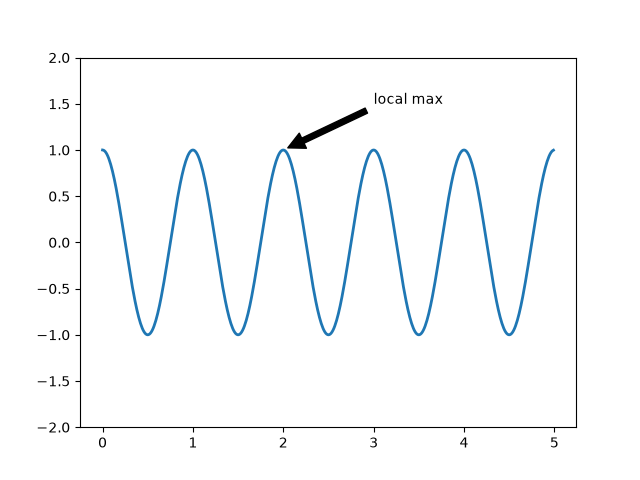## 子图 subplot

subplot(410+i) 表示子图 4x1 排列，即 4 行 1 列。此处的循环，令 i 分分别等于 1 2 3 4。

# python 相关

## 在图中使用其它字体

https://stackoverflow.com/a/53645383

/public/home/yao1/packages_ZY/anaconda_ZY/anaconda3/lib/python3.6/site-packages/matplotlib/mpl-data/fonts/ttf

rm -rf ~/.cache/matplotlib/

solution：

# 范例

]]>
<p>使用 matplotlib 绘图</p>

controlDict 中加入如下代码：

timeActivatedFileUpdate 这个功能还可以用于其他文件，请自行探索。

OpenFOAM 自带的 timeActivatedFileUpdate 不支持发动机算例。

]]>
<p>自适应输出间隔</p>
OpenFOAM Learning Resources https://openfoam.top/studyMaterials/ 2019-05-28T00:00:00.000Z 2020-04-07T00:00:00.000Z I think this is the most comprehensive learning resources about OpenFOAM.

# Books

1. The finite volume method in computational fluid dynamics : an advanced introduction with OpenFOAM® and Matlab®
2. Mathematics, Numerics, Derivations and OpenFOAM®
3. The OpenFOAM Technology Primer
4. The OpenFOAM Technology Primer
5. Getting Started with OpenFOAM® Technology: RAW
6. Jasak’s Phd thesis
7. Algorithm on Solvers and Models in OpenFOAM

# Others

download1

download2

]]>
<p>I think this is the most comprehensive learning resources about OpenFOAM.</p>
OpenFOAM 中的字典 https://openfoam.top/dictionary/ 2019-04-26T00:00:00.000Z 2020-07-13T00:00:00.000Z OpenFOAM 中的 dictionary 和 IOdictionary

# 常见用法

## 通过字典读取变量

• 为什么需要 readScalar 才能使用 lookup 读取标量？可能是因为 ITstream 无法隐式的转换成 scalar
• 为什么 lookupOrDefault 不给定模板也可以呢？因为它的第二个参数的类型就是模板的类型啊。

# 代码解析## 一些函数探秘

parent 返回的是上一级字典。
topDict 返回的是最顶层的字典。

tokens 就是这个字典中的所有元素，这里有 12 个元素，包括字符串和标点符号。
tockeys 一样的？他们的定义如下：

# 踩过的坑

## 解决方案

]]>
<p>OpenFOAM 中的 dictionary 和 IOdictionary</p>
OpenFOAM 中的喷雾速度计算 https://openfoam.top/sprayVelocity/ 2019-04-24T00:00:00.000Z 2019-11-27T00:00:00.000Z 从数学公式到 C++ 代码分析喷雾粒子速度计算，以及喷雾引起的气相速度源项

Niklas Nordin 博士论文中的公式：

$m_p \dfrac{d \mathbf{u}_p}{d t}=\mathbf{F_p}$

$\mathbf{F_p}=-\dfrac{\pi D^{2}}{8} \rho C_D\left|\mathbf{u}_{p}-\mathbf{u}\right|\left(\mathbf{u}_{p}-\mathbf{u}\right)$

$C_D=\left\{\begin{array}{ll}{\dfrac{24}{Re_{p}}\left(1+\dfrac{1}{6} R e_{p}^{2 / 3}\right)} & {Re_p<1000} \\ {0.424} & {Re_p>1000}\end{array}\right.$

$Re_{p}=\dfrac{\rho\left|\mathbf{u}_{p}-\mathbf{u}\right| d_p}{\mu_g}$

$a_p=\dfrac{d \mathbf{u}_{p}}{d t}=-\dfrac{\mathbf{u}_{p}-\mathbf{u}}{\tau_{\mathbf{u}}}$

$\tau_{\mathrm{u}}=\dfrac{8 m_{p}}{\pi \rho C_D d_p^{2}\left|\mathbf{u}_{p}-\mathbf{u}\right|}=\dfrac{4}{3} \dfrac{\rho_{p} d_p}{\rho C_{p}\left|\mathbf{u}_{p}-\mathbf{u}\right|}$

$\begin{array}{ll}\dfrac{d \mathbf{u}_{p}}{d t}\\=-\dfrac{\mathbf{u}_p-\mathbf{u}}{\tau_u}\\=-\dfrac{\mathbf{u}_p-\mathbf{u}}{\dfrac{4}{3} \dfrac{\rho_{p} d_p}{\rho C_{p}\left|\mathbf{u}_{p}-\mathbf{u}\right|}}\\=-\dfrac{3(\mathbf{u}_p-\mathbf{u})\rho C_D \left|\mathbf{u}_{p}-\mathbf{u}\right|}{4 \rho_p d_p}\\=-\dfrac{3(\mathbf{u}_p-\mathbf{u})\mu_g C_D}{4\rho_p D^2}\dfrac{\rho\left|\mathbf{u}_{p}-\mathbf{u}\right| d_p}{\mu_g}\\=-\dfrac{3}{4}\dfrac{\mu_g C_D Re_p}{\rho_p d_p^2}(\mathbf{u}_{p}-\mathbf{u})\end{array}$

$F_p = m_p \dfrac{d \mathbf{u}_p}{d t}$

$=m_p \dfrac{3}{4}\dfrac{\mu_g C_D Re_p}{\rho_p d_p^2}(\mathbf{u}-\mathbf{u}_{p})$

$=\dfrac{3m_p\mu_gC_DRe}{4\rho_p d_p^2}(\mathbf{u}-\mathbf{u}_{p})$

ReactingParcel::calc 中调用：

KinematicParcel::calcVelocity

forces.calcCoupledforces.calcNonCoupled 都定义于 ParticleForce 中，但是返回值是 0，在其派生类中重新定义真正的函数，当我们选用 SphereDragForce 时，返回值是 $\dfrac{3m_p\mu C_DRe}{4\rho d_p^2}$

Fcp$\dfrac{3m_p\mu C_DRe}{4\rho_p d_p^2}$
Fncp$0$
massEff$m_p$
acp$\mathbf{u}_{c}$ Fcp$/m_p=\dfrac{3 \mathbf{u}_{c} \mu C_D Re}{4\rho_p d_p^2}$
ancp0
bcpFcp$/m_p$
deltaU$\dfrac{\text{Fcp}}{m_p}(\mathbf{u_c}-\mathbf{u_p})dt=\dfrac{3\mu C_DRe}{4\rho_p d_p^2}(\mathbf{u_c}-\mathbf{u_p})dt$
deltaUncp$0$
deltaUcpdeltaU
dUTrans$-m_d*$deltaU
Spudt*Fcp

KinematicCloud::SU

ReactingParcel::calc 中：

$\frac{\partial \overline{\rho} \tilde{u}_{i}}{\partial t}+\frac{\partial \overline{\rho} \tilde{u}_{i} \tilde{u}_{j}}{\partial x_{j}}=-\frac{\partial \overline{p}}{\partial x_{i}}+\frac{\partial \overline{\tau}_{i j}}{\partial x_{j}}-\frac{\partial \overline{\rho} \Gamma_{i j}}{\partial x_{j}}+\overline{\dot{S}}_i$

$\overline{\dot{S}}_{i}=\frac{1}{V_{f}} \sum_{m=1}^{n p} \left(n_{d, m} \dot{m}_{d, m} u_{d, i, m}-n_{d, m} F_{d, i, m}\right)$

SU.source()$=-(n_d\dot m u_d - F)$

]]>
<p>从数学公式到 C++ 代码分析喷雾粒子速度计算，以及喷雾引起的气相速度源项</p>
OpenFOAM 速度输运方程中的应力张量项 https://openfoam.top/shearRateTensor/ 2019-04-21T00:00:00.000Z 2019-04-22T00:00:00.000Z 无摘要

## 速度输运方程

$\frac{ \partial }{ \partial t }( \bar\rho\widetilde{u_i} ) + \frac{ \partial\bar\rho\widetilde{u_i}\widetilde{u_j} }{ \partial x_j } = \frac{ \partial \overline \sigma _{ij} }{ \partial x_j } + \frac{ \partial }{ \partial x_j}( -\bar\rho\widetilde{ u'_iu'_j } )$

$\overline \sigma _{ij} = \overline\tau_{ij} - \overline p\delta_{ij} = 2\mu( \overline S_{ij} - \frac{1}{3}\overline S_{kk}\delta_{ij} ) - \overline p\delta_{ij}$

$\Gamma_{ij}=-\bar\rho \widetilde {u'_iu_j'}$

$\begin{array}{ll}&-\nabla\cdot\left(\rho*\nu_{eff}\left((\nabla\otimes\mathbf{u})^T-\dfrac{2}{3}tr(\nabla\otimes\mathbf{u})^T\right)\right)\\&-\nabla\cdot(\rho*\nu_{eff}\nabla\otimes\mathbf{u})\\&=-\nabla\cdot\left( \rho*\nu_{eff}(\nabla\otimes\mathbf{u}+(\nabla\otimes\mathbf{u})^T)-\dfrac{2}{3}\rho*\nu_{eff} tr(\nabla\otimes\mathbf{u})^T\right)\\&=-\nabla\cdot\left( \rho*\nu_{eff}(\nabla\otimes\mathbf{u}+(\nabla\otimes\mathbf{u})^T)-\dfrac{2}{3}\rho*\nu_{eff}(\nabla \cdot \mathbf{u})\mathbf{I}\right)\\&=-\nabla\cdot\left( 2\rho*\nu_{eff}S_{ij}-\dfrac{2}{3}\rho*\nu_{eff}S_{kk}\delta_{ij}\right)\\&=-\nabla\cdot\left( 2\rho*(\nu+\nu_t)S_{ij}-\dfrac{2}{3}\rho*(\nu+\nu_t)S_{kk}\delta_{ij}\right)\\&=-\nabla\cdot(\tau_{ij}+\Gamma_{ij})\end{array}$

$\nabla\otimes\mathbf{u}= \left(\begin{matrix}\dfrac{\partial u}{\partial x}&\dfrac{\partial u}{\partial y}&\dfrac{\partial u}{\partial z}\\\dfrac{\partial v}{\partial x}&\dfrac{\partial v}{\partial y}&\dfrac{\partial v}{\partial z}\\\dfrac{\partial w}{\partial x}&\dfrac{\partial w}{\partial y}&\dfrac{\partial w}{\partial z}\end{matrix} \right)$

$(\nabla\otimes\mathbf{u})^T=\left(\begin{matrix}\dfrac{\partial u}{\partial x}&\dfrac{\partial v}{\partial x}&\dfrac{\partial w}{\partial x}\\\dfrac{\partial u}{\partial y}&\dfrac{\partial v}{\partial y}&\dfrac{\partial w}{\partial y}\\\dfrac{\partial u}{\partial z}&\dfrac{\partial v}{\partial z}&\dfrac{\partial w}{\partial z}\end{matrix} \right)$

$tr(\nabla\otimes\mathbf{u})=tr\left((\nabla\otimes\mathbf{u})^T\right)=\dfrac{\partial u_k}{\partial x_k}=\nabla \cdot \mathbf{u}=tr(S_{ij})$

strain rate $S_{ij}$ :

$S_{ij}=\frac{1}{2}( \frac{ \partial u_i }{ \partial x_j } + \frac {\partial u_j }{ \partial x_i } )=\dfrac{1}{2}\left(\nabla\otimes\mathbf{u}+(\nabla\otimes\mathbf{u})^T\right)$

$S_{ij}=\left(\begin{matrix}\dfrac{\partial u}{\partial x}&\dfrac{1}{2}(\dfrac{\partial u}{\partial y}+\dfrac{\partial v}{\partial x})&\dfrac{1}{2}(\dfrac{\partial u}{\partial z}+\dfrac{\partial w}{\partial x})\\S_{21}&\dfrac{\partial v}{\partial y}&\dfrac{1}{2}(\dfrac{\partial v}{\partial z}+\dfrac{\partial w}{\partial y})\\S_{31}&S_{32}&\dfrac{\partial w}{\partial z}\end{matrix} \right)$

$S_{ij}$ 是对称矩阵，$S_{12}=S_{21},\ S_{13}=S_{31},\ S_{23} = S_{32}$

$tr(S_{ij})=S_{kk}=\dfrac{\partial u_k}{\partial x_k}=\nabla\cdot \mathbf{u}$

$S_{ij}$ 在代码中是：symm(fvc::grad(U))，其中 symm(tensor) = 1/2*(tensor + T(tensor))

deformation rate tensor $D_{ij}$:

$\begin{array}{ll}&D_{ij}= S_{ij}-\frac{1}{3}tr(S_{ij})\\&=\left( \begin{matrix} S_{11}-\frac{1}{3}(S_{11}+S_{22}+S_{33}) & S_{12} & S_{13} \\ S_{21} & S_{22}-\frac{1}{3}(S_{11}+S_{22}+S_{33}) & S_{23} \\ S_{31} & S_{32} & S_{33}-\frac{1}{3}(S_{11}+S_{22}+S_{33}) \end{matrix} \right) \\&=S_{ij}-\frac{1}{3}\nabla\cdot \mathbf{u} \delta_{ij}\end{array}$

$D_{ij}$ 在代码中是：dev(symm(fvc::grad(U)))，也就是 dev(S_{ij})

Tobias Holzmann. Mathematics, Numerics, Derivations and OpenFOAM®, Holzmann CFD,
URL www.holzmann-cfd.de, DOI: 10.13140/RG.2.2.27193.36960.

Cauchy stress tensor $\mathbf{\sigma}=\mathbf{\sigma}=-p\mathbf{I}+[\mathbf{\sigma}-\frac{1}{3}tr(\mathbf{\sigma})\mathbf{I}]$

shear−rate tensor $\mathbf{\tau}=\mathbf{\sigma}-\frac{1}{3}tr(\mathbf{\sigma})\mathbf{I}=\mathbf{\sigma}^{dev}$

Reynolds-Stress tensor
$\overline \mathbf{\sigma}_t=-\bar\rho \widetilde {u'_iu_j'}$

Boussinesq 假设：

$\overline \mathbf{\sigma}_t-\frac{1}{3}tr(\overline \mathbf{\sigma}_t)=2\mu_t \bar \mathbf{D}-\frac{2}{3}tr(\bar \mathbf{D})\mathbf{I}$

$tr(\overline \mathbf{\sigma}_t)=tr(-\bar\rho \widetilde {u'_iu_j'})=(-\bar\rho \widetilde {u'u'})+(-\bar\rho \widetilde {v'v'})+(-\bar\rho \widetilde {w'w'})=-2\bar\rho \mathbf{I}k$

$\overline \mathbf{\sigma}_t=2\mu_t \bar \mathbf{D}-\frac{2}{3}tr(\bar \mathbf{D})\mathbf{I}+\frac{1}{3}tr(\overline \mathbf{\sigma}_t)=2\mu_t \bar \mathbf{D}-\frac{2}{3}tr(\bar \mathbf{D})\mathbf{I}-\frac{2}{3}\bar\rho \mathbf{I}k$

$tr(\overline \mathbf{\sigma}_t)=tr(\overline \mathbf{\tau}_t-\frac{2}{3}\bar\rho \mathbf{I}k)=-2\bar\rho \mathbf{I}k\\tr(\overline \mathbf{\sigma}_t)=tr(2\mu_t\bar \mathbf{D}-\frac{2}{3}\mu_ttr(\mathbf{D})\mathbf{I}-\frac{2}{3}\bar\rho \mathbf{I}k)=-2\bar\rho \mathbf{I}k$

## 对于 RANS

$\frac{ \partial }{ \partial t }( \bar\rho\widetilde{u_i} ) + \frac{ \partial\bar\rho\widetilde{u_i}\widetilde{u_j} }{ \partial x_j } = \frac{ \partial \overline \sigma _{ij} }{ \partial x_j } + \frac{ \partial }{ \partial x_j}( \Gamma_{ij} )$

$\frac{ \partial }{ \partial t }( \bar\rho\widetilde{u_i} ) + \frac{ \partial\bar\rho\widetilde{u_i}\widetilde{u_j} }{ \partial x_j } = -\frac{\partial p}{\partial x_i}-\frac{\partial}{\partial x_j}\left(\frac{2}{3}\bar\rho \delta_{ij}k\right)+\frac{\partial}{\partial x_j}\left[2(\mu+\mu_t)(S_{ij}-\frac{\partial u_k}{\partial x_k}\delta_{ij})\right]$

OF 速度输运方程代码里边并没有包含 $k$ 这一项，因此代码里的 $p$ 并不是真正的压力，而是 $p+\frac{2}{3}\rho k$。Page 76 in the book of Tobias.

## 对于 LES

$\frac{ \partial }{ \partial t }( \bar\rho\widetilde{u_i} ) + \frac{ \partial\bar\rho\widetilde{u_i}\widetilde{u_j} }{ \partial x_j } = \frac{ \partial \overline \sigma _{ij} }{ \partial x_j } - \frac{ \partial }{ \partial x_j}( \Gamma_{ij} )$

Boussinesq 假设：

$\Gamma_{ij}-\frac{1}{3}\Gamma_{kk}\delta_{ij}=-2\mu_t(\widetilde{S_{ij}}-\frac{1}{3}\widetilde{S_{kk}}\delta_{ij})$

LES 中有 $tr(\Gamma_{ij})=\Gamma_{kk}=2\bar\rho k$

$\Gamma_{ij}=-2\mu_t(\widetilde{S_{ij}}-\frac{1}{3}\widetilde{S_{kk}}\delta_{ij})-\frac{2}{3}\bar\rho k \delta_{ij}$

$\frac{ \partial }{ \partial t }( \bar\rho\widetilde{u_i} ) + \frac{ \partial\bar\rho\widetilde{u_i}\widetilde{u_j} }{ \partial x_j } = -\frac{\partial p}{\partial x_i}+\frac{\partial}{\partial x_j}\left(\frac{2}{3}\bar\rho \delta_{ij}k\right)+\frac{\partial}{\partial x_j}\left[2(\mu+\mu_t)(S_{ij}-\frac{\partial u_k}{\partial x_k}\delta_{ij})\right]$

https://www.cfd-online.com/Forums/openfoam-solving/58214-calculating-divdevreff.html

## 一些矢量和张量的操作

LES 中经常出现的 $|\tilde S|=\sqrt{2\widetilde{S_{ij}}\widetilde{S_{ij}}}$，用代码表示是：

& 是内积，也叫数量积，也叫点乘，两个矢量的内积是一个标量。
^ 是叉乘，两个矢量的叉乘，仍旧是一个矢量。在三维几何中，向量a和向量b的叉乘结果是一个向量，更为熟知的叫法是法向量，该向量垂直于a和b向量构成的平面。

$sqr(U)=u_iu_j$

]]>
<p>无摘要</p>
fvMesh 简单分析（待补充） https://openfoam.top/fvMesh/ 2019-04-14T00:00:00.000Z 2019-04-16T00:00:00.000Z 无摘要primitiveMesh没有更顶层的类了。常用的方法：

surfaceInterpolation没有更顶层的类了。

lduMesh没有更顶层的类了。

fvMesh 常用的方法：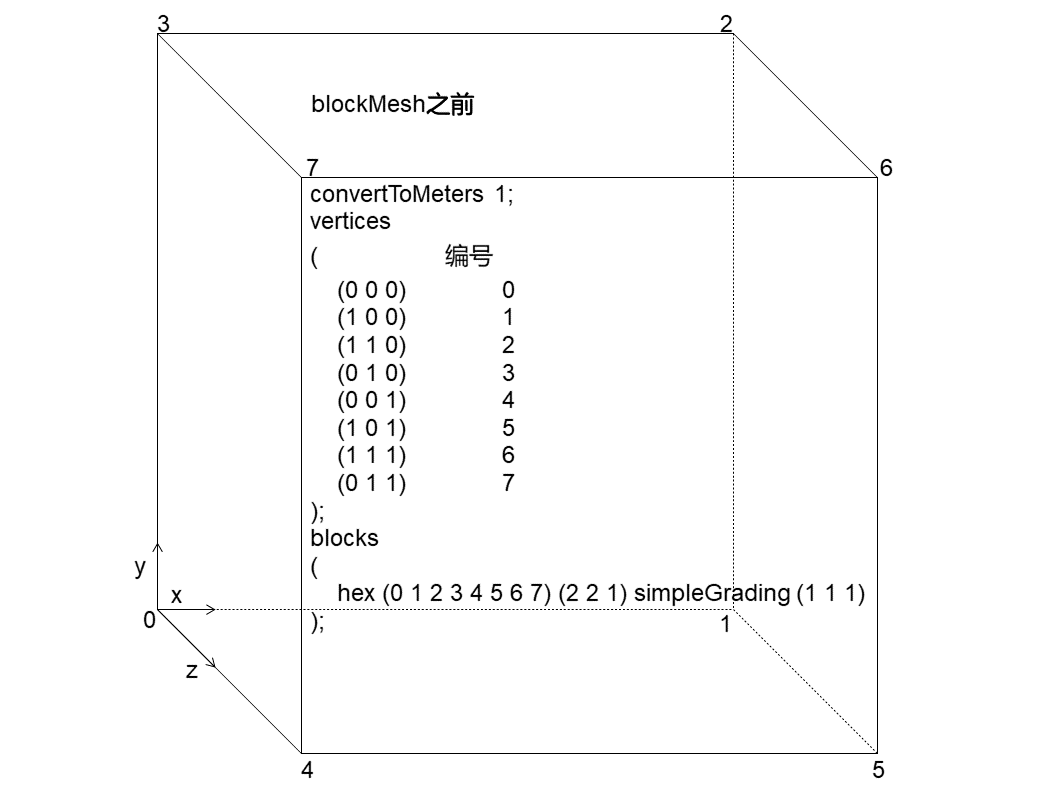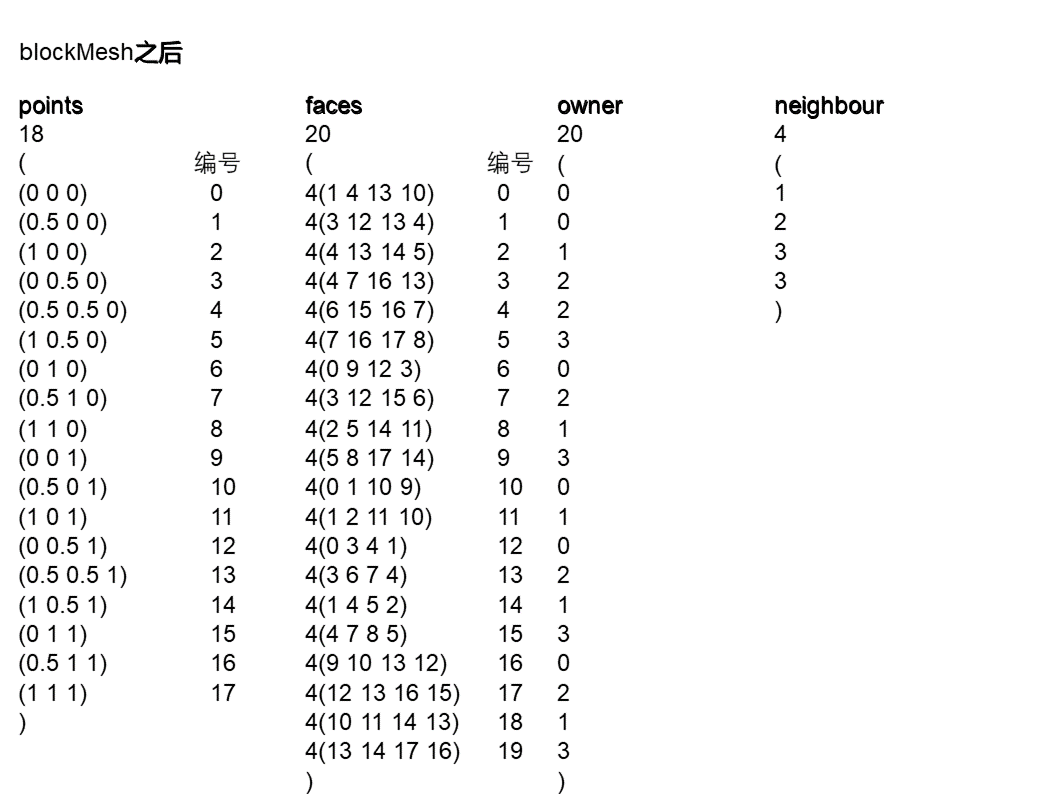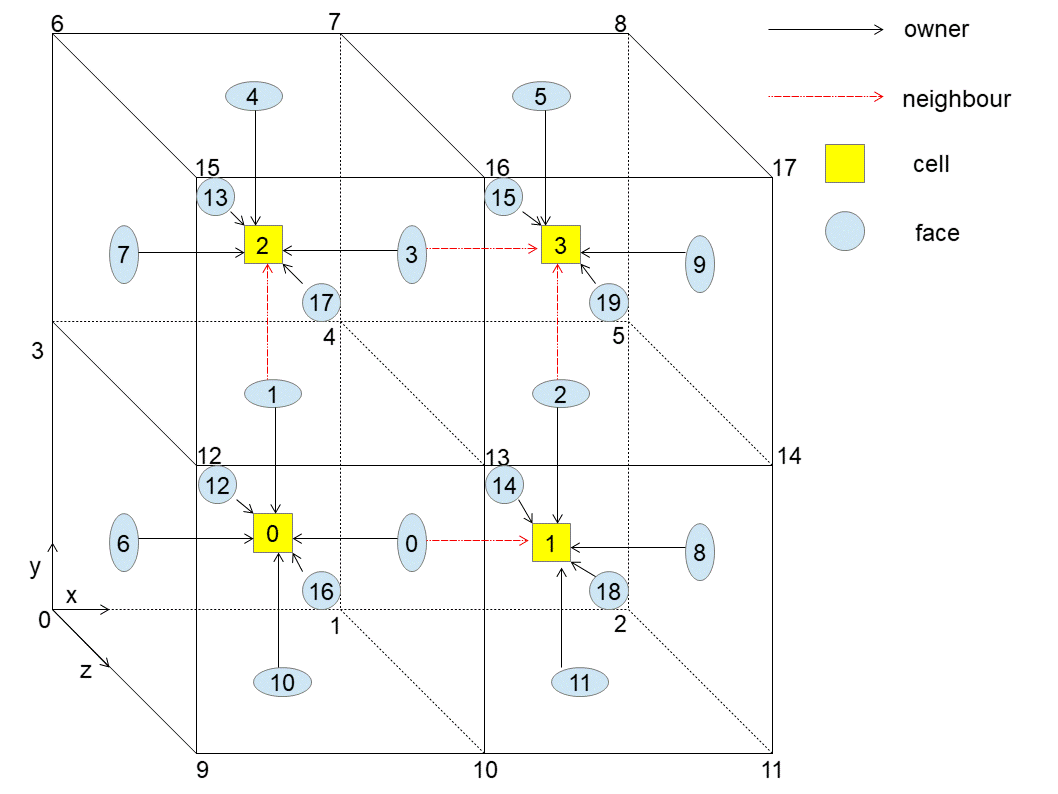]]>
<p>无摘要</p>
OpenFOAM 场（field）的操作和运算 https://openfoam.top/fields/ 2019-04-01T00:00:00.000Z 2021-02-16T00:00:00.000Z Field Operation And Manipulation

# field 相关类的结构GeometricField 类定义于 src\OpenFOAM\fields\GeometricFields\GeometricField

• GeometricField – 场 + 网格，包含内部场及其边界场，边界场是场的场（FieldField）
• DimensionedField – 带单位的场，只有内部场，没有边界场
• Field – 数组（即 List）+ 代数操作

# field 相关类的用法

## 构造

0.*A 调用的是定义于 GeometricFieldFunctionsM.C 中的这个函数

## 获取

• const

• const Internal& internalField() const, 有量纲的内部场
• const typename Internal::FieldType& primitiveField() const, 无量纲的内部场
• const Boundary& boundaryField() const, 无量纲的边界场
• non-const

• Internal& ref(), 有量纲的内部场
• typename Internal::FieldType& primitiveFieldRef(), 无量纲的内部场
• Boundary& boundaryFieldRef(), 无量纲的边界场

forAll 来遍历的时候，遍历的其实就是那个 field<Type>

T 文件：

# 场的操作和运算 (Field Operation And Manipulation)

• writeOpt
使用举例：

• 平均
• 加权平均

• dimensions
使用举例：

## GeometricField 中定义的函数

• max, min
取最大值，包括内部场和边界场。并行计算时，Info 输出的是 master 的还是全局的？未测试
使用举例：
• writeMinMax
输出最小最大值，只包括内部场。

T 文件：

TFOR_ALL_F_OP_FUNC_S_F 和 TFOR_ALL_F_OP_FUNC_F_S 定义于 FieldM.H

MAXMIN 定义于 int.H

FieldFunctions.C 中还定义了几个诸如此类的函数

• gMax
max 的并行版，但是返回的是无量纲的值？

## fvc 中的操作

### volumeIntegrate

volumeIntegrate 的功能是对每个网格的某个物理量，乘以其网格体积，然后形成一个新的场。

### domainIntegrate

domainIntegrate 的功能是对某个物理量，乘以其网格体积，然后对所有网格求和。

]]>
<p><strong>F</strong>ield <strong>O</strong>peration <strong>A</strong>nd <strong>M</strong>anipulation</p>